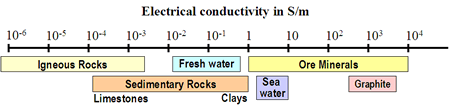# Conductivity¶

Electromagnetic methods are sensitive principally to electrical conductivity $$\sigma$$ (Siemens/meter). We sometimes work with the inverse of conductivity which is electrical resistivity $$\rho$$ which has units $$\Omega m$$ (ohm- meters). Electrical conductivity characterizes the ease that current flows through the material when an electrical force is applied. Electric current (units of Amperes) quantifies the amount of charge that is moving by an observer in one second. The electrical conductivity of Earth’s materials varies over many orders of magnitude. It depends upon many factors, including: rock type, porosity, connectivity of pores, nature of the fluid, and metallic content of the solid matrix. A very rough indication of the range of conductivity for rocks and minerals is presented in Fig. 140.While conductivity is the generally the main physical property of interest, EM induction methods also depend on the magnetic permeability and electrical permitivity of rocks. Their importance depends upon the frequency of the EM signal used for given a system.